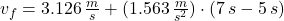## A hockey puck with mass 0.160 kg is at rest at the origin (x=0) on the horizontal, frictionless surface of the rink. At time t = 0 a player

Question

A hockey puck with mass 0.160 kg is at rest at the origin (x=0) on the horizontal, frictionless surface of the rink. At time t = 0 a player applies a force of 0.250 N to the puck, parallel to the x-axis; he continues to apply this force until t = 2.00s.

What is the position of the puck at t = 2.00s ?
In this case what is the speed of the puck?
If the same force is again applied at t = 5.00s , what is the position of the puck at t = 7.00s ?
In this case what is the speed of the puck?

in progress 0
6 months 2021-08-27T21:13:51+00:00 1 Answers 0 views 0

a)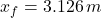, b)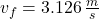, c)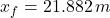, d)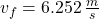Explanation:

a) Position of the puck:

The acceleration experimented by the puck is: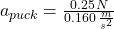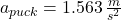As the force remains constant during its time of application, accelaration is also constant. Position at given time is the following: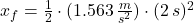b) Speed of the puck:

The speed of the puck is computed as follows: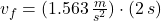c) The absence of external force and the fact that ground is frictionless lead to the conclusion that hockey puck moves out at constant speed from 2 s. to 5 s. Then, the initial speed is: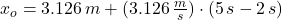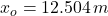Likewise, the initial speed is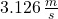.

The new application of the same force means the return of a accelerated movement. Then: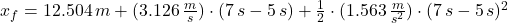d) The final speed of the puck is: